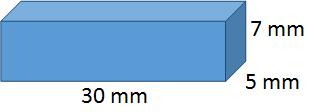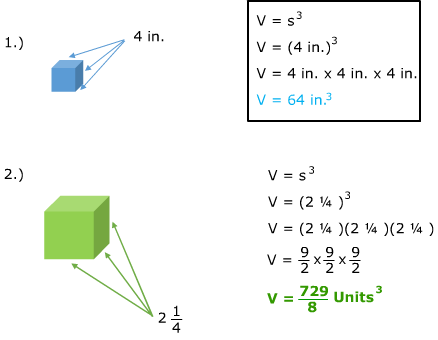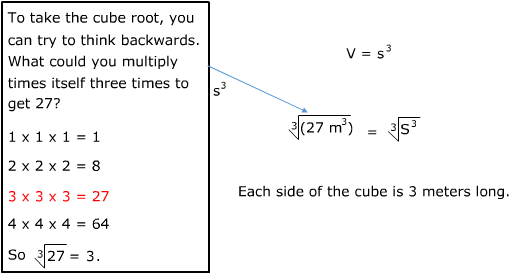# Volume of a Cube

Recall that the volume of a rectangular prism can be found by multiplying the length, width and height.V = l w h

V = (30 mm)(5 mm)(7 mm)

V = 1050 mm3

Take a look at an example of a special type of rectangular prism, where all of the sides are the same length. We call this prism a cube.We can simplify the formula for cubes. Because the length, width and height are all the same, we can use V = s3 instead.

V = l w h     V = s3

V = (5 mm)(5 mm)(5 mm)    V = (5 mm)3

V = 125 mm3  V = 125 mm3

Notice that the answers are the same no matter which method you use. However, the second formula can help to simplify the process.

Try a few more:If you have a cube and know the volume, you can also use this formula to determine the length of each side of the cube.

3.) Determine the side length of a cube with a volume of 27m3.4.) Determine the side length of a cube with a volume of 1953.125 in.3Let's Review

All of the sides of a cube are the same length. To calculate the volume of a cube using the formula V = l w h or simplify it to say V = s3. If you know the volume of a cube you can determine the length of the sides by calculating the cube root.